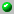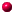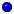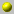Anatol N. Kirillov

The homepage can be accessed here.

Anatol N. Kirillov

Nagoya University
Chikusa-ku, Nagoya 486-8602
Japan

phone: 81-(52)-789-5605
fax: 81-(52)-789-2829
e-mail: kirillov@math.nagoya-u.ac.jp

the following papers are available on our server= dvi format= ps format= tex format= pdf formata_bijection_between_littlewood_richardson_tableaux_and_rigged_configurationsaffine_hecke_algebras_and_raising_operators_for_macdonald_polynomialsa_generalization_of_kostka_foulkes_polynomialsberenstein_zelevinsky_triangles_elementary_couplings_and_fusion_rulescan_fusion_coefficients_be_calculated_from_the_depth_rulecan_fusion_coefficients_be_calculated_from_the_depth_rulecan_fusion_coefficients_be_calculated_from_the_depth_rulecauchy_identities_for_universal_schubert_polynomialscharacter_formulae_of_sl_n_modules_and_inhomogenous_paths
combinatorial_b_n_analogues_of_schubert_polynomialsdomino_tableaux_schuetzenberger_involuition_and_the_symmetric_group_actiondomino_tableaux_schuetzenberger_involuition_and_the_symmetric_group_action_v2
new_combinatorial_formula_for_modified_hall_littlewood_polynomialsnew_combinatorial_formula_for_modified_hall_littlewood_polynomialson_some_quadratic_algebrasquadratic_algebras_dunkl_elements_and_schubert_calculusquantum_grothendieck_polynomialsquantum_schubert_polynomials_and_quantum_schur_functionsquantum_schubert_polynomials_and_the_vafa_intriligator_formulaskew_divided_difference_operators_and_schubert_polynomialst_deformations_of_quantum_schubert_polynomialsunimodality_of_principal_spezialization_of_the_internal_product_of_the_schur_functionsuniversal_exponential_solution_of_the_yang_baxter_equationvarious_representations_of_the_generalized_kostka_polynomialsyang_baxter_equation_symmetric_functions_and_grothendieck_polynomials

Go back to the directory of pre and reprints.
Or search the database by keywords
Or look for the newest entires in the database